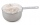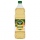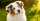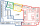# Calculator What is 3/4 as a mixed number

The calculator performs basic and advanced operations with mixed numbers, fractions, integers, decimals. Mixed fractions are also called mixed numbers. A mixed fraction is a whole number and a proper fraction combined, i.e. one and three-quarters. The calculator evaluates the expression or solves the equation with step-by-step calculation progress information. Solve problems with two or more mixed numbers fractions in one expression.

### 3/4 = 3/4 = 0.75

Spelled result in words is three quarters.

## Mixed fractions in word problems:

• Zailene 2Zailene has 8 2/3 cup of flour. She used 5 1/2 cups of flour. How many cups of flour was left to her?
• A more than bWhat is 5 1/7 more than 2 3/7?
• Mixed2improperWrite the mixed number as an improper fraction. 166 2/3Add this two mixed numbers: 1 5/6 + 2 2/11=
• A scienceA science experiment calls for making 3 and 2/3 cups of distilled water with 1 and 3/5 cups of vinegar and 2/3 cups of liquid detergent how much liquid in all and cups is needed?
• Difference mixed fractionsWhat is the difference between 4 2/3 and 3 1/6?
• A fullA full bag of dog food weights 5 1/2 pounds. How much dog food do you have if there are 3 3/4 bags in the cupboard?
• The product 3The product of two numbers is 15 ⁵/₆. If one of the numbers is 5 ²/₃, find the other.
• Sum of mixedThe sum of two mixed numbers is 15½. One of the number is 5 ¾, what is the other?
• Jack plantedJack planted 1 1/4 rows of lettuce in the family garden, Rose planted 1 1/2 rows of broccoli and Calvin planted 4 5/12 rows of beans. How many rows were planted in the garden?
• An electricianAn electrician needs 1 1/3 rolls of electrical wire to wire each room in a house. How many rooms can he wire with 6 2/3 rolls of wire?
• Original numberJoe divided a number by 2 1/4 to get 2/3. What was the original number?
• Perimeter - rectangleFind the perimeter of the rectangle. A rectangle with a base of (3 5/6) m and a height of (2 3/7) m Its perimeter is x= meters. (Use fraction in your answer. )Add this two mixed numbers: 1 5/6 + 2 2/11=Add two mixed fractions: 2 4/6 + 1 3/62 and 1/8th plus 1 and 1/3rd =A number, X, is subtracted from 8 1/4. The result is 12 3/5. What is the value of X?